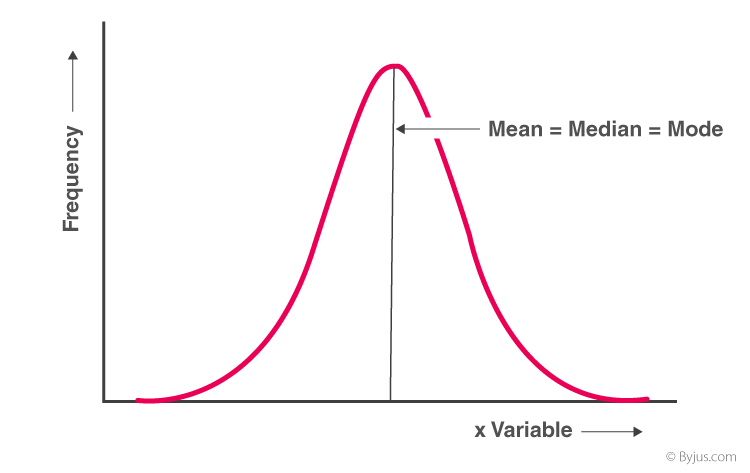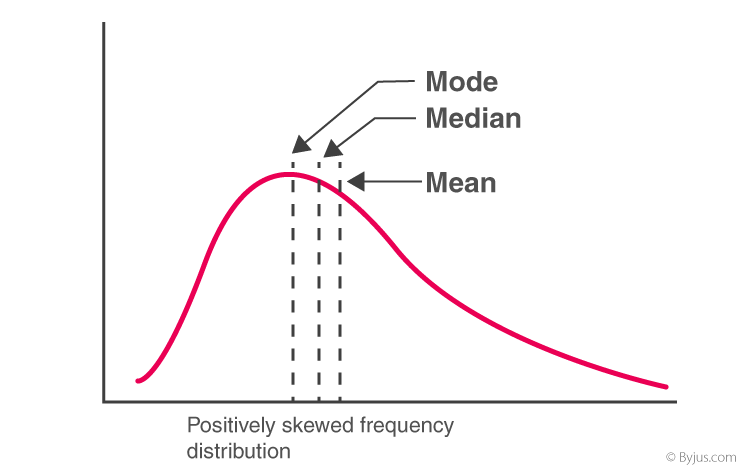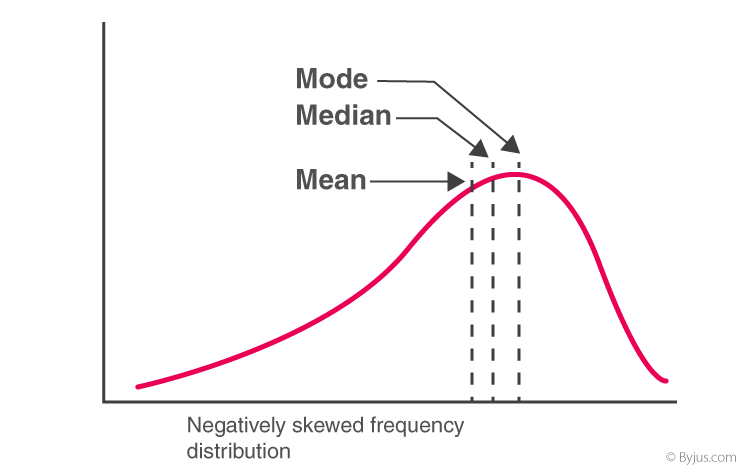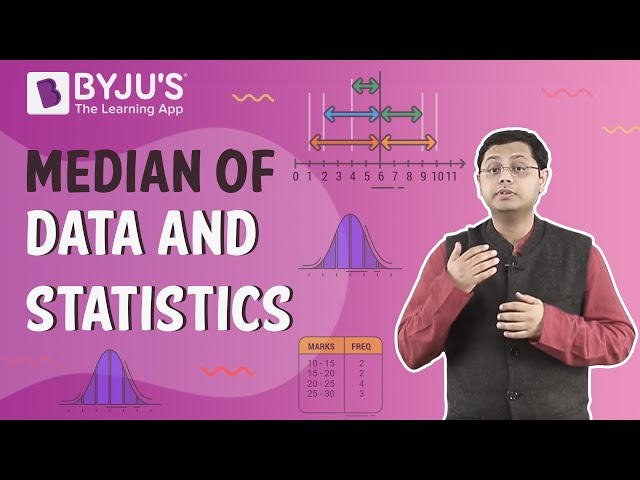# Relation Between Mean Median and Mode

In statistics, for a moderately skewed distribution, there exists a relation between mean, median and mode. This mean median and mode relationship is known as the empirical relationship” which is defined as Mode is equal to the difference between 3 times the median and 2 times the mean. This relation has been discussed in detail below.

To recall,

• Mean is the average of the data set which is calculated by adding all the data values together and dividing it by the total number of data sets.
• Median is the middle value among the observed set of values and is calculated by arranging the values in ascending order or in descending order and then choosing the middle value.
• Mode is the number from a data set which has the highest frequency and is calculated by counting the number of times each data value occurs.

## Empirical Relationship between Mean, Median and Mode

In case of a moderately skewed distribution, the difference between mean and mode is almost equal to three times the difference between the mean and median. Thus, the empirical mean median mode relation is given as:

 Mean – Mode = 3 (Mean – Median)

Or

 Mode = 3 Median – 2 Mean

Either of these two ways of equations can be used as per the convenience since by expanding the first representation we get the second one as shown below:

Mean – Mode = 3 (Mean – Median)

Mean – Mode = 3 Mean – 3 Median

By rearranging the terms,

Mode = Mean – 3 Mean + 3 Median

Mode = 3 Median – 2 Mean

However, we can define the relation between mean, median and mode for different types of distributions as explained below:

### Mean Median Mode Relation With Frequency Distribution

• Frequency Distribution with Symmetrical Frequency Curve

If a frequency distribution graph has a symmetrical frequency curve, then mean, median and mode will be equal.Mean = Median = Mode
• For Positively Skewed Frequency Distribution

In case of a positively skewed frequency distribution, the mean is always greater than median and the median is always greater than the mode.Mean > Median > Mode
• For Negatively Skewed Frequency Distribution

In case of a negatively skewed frequency distribution, the mean is always lesser than median and the median is always lesser than the mode.Mean < Median < Mode

Also Check:
Mean Median Mode Formula

### Example Question Using the Mean, Median and Mode Relationship

Question: In a moderately skewed distribution, the median is 20 and the mean is 22.5. Using these values, find the approximate value of the mode.

Solution:

Given,

Mean = 22.5

Median = 20

Mode = x

Now, using the relationship between mean mode and median we get,

(Mean – Mode) = 3 (Mean – Median)

So,

22.5 – x = 3 (22.5 – 20)

22.5 – x = 7.5

∴ x = 15

So, Mode = 15.

## Video Lesson on Median of DataKeep visiting BYJU’S to learn more such different maths articles. Also, register now to download various maths materials like sample papers, question papers, NCERT solutions and get several video lessons to learn more effectively.

## Frequently Asked Questions – FAQs

Q1

### What is the meaning of mean, median and mode?

For any given data, mean is the average of given data values and this can be calculated by dividing the sum of all data values by number of data values. Median is the middlemost value of the data set when data values are arranged either in ascending or descending order. Mode is the most frequently occurred data value.
Q2

### What is empirical relationship between mean median and mode?

Empirical relationship between mean median and mode for a moderately skewed distribution can be given as:
Mean – Mode = 3 (Mean – Median)
Or
Mode = 3 Median – 2 Mean
Q3

### What is the relation between mean median and mode for a frequency distribution with symmetrical frequency curve?

For a frequency distribution with symmetrical frequency curve, the relation between mean median and mode is given by:
Mean = Median = Mode
Q4

### What is the relation between mean median and mode for a positively skewed frequency distribution?

For a positively skewed frequency distribution, the relation between mean median and mode is:
Mean > Median > Mode
Q5

### What is the relation between mean median and mode for a negatively skewed frequency distribution?

For a negatively skewed frequency distribution, the relation between mean median and mode is:
Mean < Median < Mode
Test your Knowledge on Relation Between Mean Median and Mode